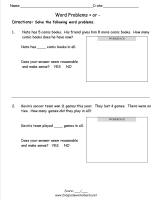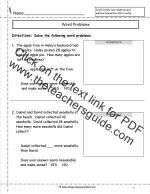﻿ Word Problems Worksheets

# Word Problem Worksheets

## Addition Word Problems Worksheets, Subtraction Word Problem Worksheets, Multiplication Word Problem Worksheets

###Addition and Subtraction Word Problems

Common Core State Standards: 2.OA.2
Operations & Algebraic Thinking
Represent and solve problems involving addition and subtraction.

Use addition and subtraction within 100 to solve one- and two-step word problems involving situations of adding to, taking from, ......

Single digit word problems.

###Addition and Subtraction Word Problems

Common Core State Standards: 2.OA.2
Operations & Algebraic Thinking
Represent and solve problems involving addition and subtraction.

Use addition and subtraction within 100 to solve one- and two-step word problems involving situations of adding to, taking from, ......

Two digit word problems.

###Multiplication Word Problems

Common Core State Standards: 3.OA.3
Operations & Algebraic Thinking
Represent and solve problems involving multiplication and division.

Use multiplication and division within 100 to solve word problems.....

Multiplication word problems.